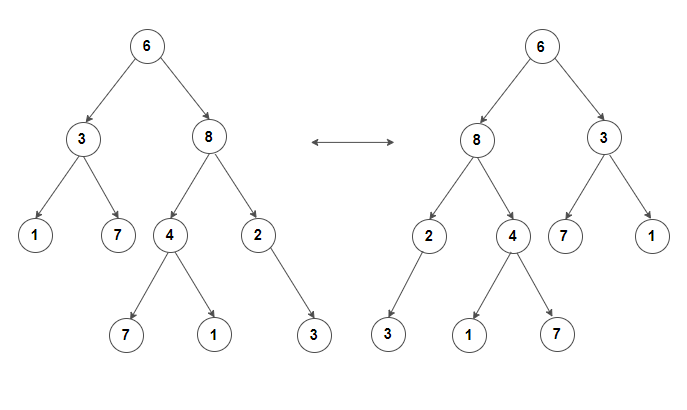# Determine if binary tree can be converted to another by doing any number of swaps of left and right child

Given a binary tree, write an efficient algorithm to determine if it can be converted to another binary tree by doing any number of swaps of its right and left branches.

For example, consider binary tree shown on the left below. It should be converted to binary tree shown on the right.We can easily solve this problem by using recursion. We return true if

1. both trees are same or both trees are empty or

2. both trees are non-empty and value of root of X and Y are same and

(a) left subtree of X can be converted to right subtree of Y and right subtree of X can be converted to left subtree of Y.

(b) right subtree of X can be converted to left subtree of Y and left subtree of X can be converted to right subtree of Y.

C++ implementation –

The time complexity of above solution is O(n) and need O(h) extra space for the call stack where h is the height of the tree.(1 votes, average: 5.00 out of 5)Loading...